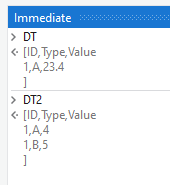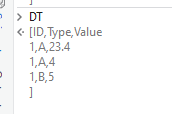# Need to append datatable if the next value is already present

I have a datatable with value as

ID. Type. VALUE

1. `````` A.       23.4
``````

In loop when I get next value which has ID as 1 and type A value 2.i should not add as new row instead i need to add as

Output
ID. Type. Value

1. `````` A.      25.4(23.4+2) ID and type should be same then only i need to append in the same row if I'd or type is different i need to add as new row
``````

Hi @sruthesanju
I got confused that u have mention ID and type is same then do in same row
Could u elaborate
Bcz
ID ->1
Type->A

Thanks
Harivishnu

Hi @sruthesanju ,

Let us know the Full details. With which data is the loop happening ? Are we looping through the Datatable that you have specified ? But it only has one row ?

We do understand that an Update needs to happen to the Datatable, But we do not know if that is the Datatable that is being looped or do we have some other Collection that we are comparing with ?

It’s not in loop i am add the value in datatable inside for loop in first loop the value of I’d type value will be added when the loop run second time if the ID and type is same as previous added in datatable need to append as i mentioned above

For loop
{

}

Thanks,
Harivishnu

Is this clear

I don’t have xanl files just i have posted my idea .i need to implement it by uipsth

Let me explain in diff way .i have an excel with data’s as

ID. TYPE. VALUE

1. `````` A.        23.4
``````

Next i am trying to update another Data in excel but the data has same ID and Type as previous value present in excel.

That another data is

ID. TYPE. VALUE

1. `````` A.         4
``````

SINCE THE I AND SECOND HAS SAME ID AND TYPE I NEED TO ADD THE VALUE COLUMN ALONE ie 23.4+4AND UPDATE IN EXCEL

So my output will be

ID. TYPE. VALUE

1. A. 27.4

What should be done if the ID and the Type are different ?

Need to update as new row

If ID IS DIFFERENT MEANS

ID TYPE VALUE

1. A. 33

NEXT I NEED TO UPDATE ID 2 AND TYPE A AND VALUE AS 4

SINCE THE ID IS DIFFERENT

UPDATE AS
ID TYPE VALUE

1. A. 33

2. A. 4

ID AND TYPE SHOULD BE SAME TO UPDATE IN EXISTING ROW OR ELSE ADD AS NEW ROW

For cases like these, maybe an approach using `Merge Datatables` Activity, Combining the Two Datatables into one at first and then performing the Grouping based on `ID` and `Type` Columns and Summing up the `Value` Column could be a Solution.

Group By and Sum Query used :

``````(From r In DT.AsEnumerable
Group By k = r("ID").ToString, a =r("Type").ToString Into grp = Group
Let ra =New Object(){k,a,grp.Sum(Function(x)CDbl(x("Value").ToString))}
``````

Debug Panel :

Inputs (DT & DT2) :Merged DT :Output DT :Let us know if this method works out for your case.

This is working fine,

I need another one scenario too

ID. TYPE. VALUE. DATE

1. ``````  A.       23.4.      12/31/2022
``````
2. ``````   A.        4.          12/31/2023
``````

IF THE INPUT IS LIKE OTHER THAN ID TYPE OTHER COLUMN SHOULD BE SEPERATE WITH COMMAS

OUTPUT SHOULD BE:

ID. TYPE. VALIE. DATE

1. ``````   A.         27.4.    12/31/2022,12/31/2023
``````

Is Date the only other column or do you have many other columns which you would need to concatenate ?

You could change the Linq query to the below for the case where Date column values are to be concatenated :

``````(From r In DT.AsEnumerable
Group By k = r("ID").ToString, a =r("Type").ToString Into grp = Group
Let ra =New Object(){k,a,grp.Sum(Function(x)CDbl(x("Value").ToString)),String.Join(",",grp.Select(Function(x)x("Date").ToString).ToArray)}
``````

Let us know if this doesn’t work.

Input DT

Since name column is same for ID 1 it need not to be added like this instead it need to be added as ABC alone

I am getting the output like this name column is empty and deposit date has “”

There was no mention of the name column or that it must not be concatenated. Do provide us with a complete requirement as to what/How many columns are present. What columns are needed to be combined, concatenated and what columns are to be left alone/Keep First instance.

Do note that providing the names of the columns would be helpful for us to configure the GroupBy clause in an approachable manner.

We do not want to go back and forth on multiple sub requirements on a Same Topic, which would make the Topic requirement unclear for others when searched.

Ok only name column will be there other than that no changes

Apologies for the change

Check with the below Updated Expression :

``````(From r In DT.AsEnumerable
Group By k = r("ID").ToString, a =r("Type").ToString Into grp = Group
Let ra =New Object(){k,a,grp.Sum(Function(x)CDbl(x("Value").ToString)),String.Join(",",grp.Select(Function(x)x("Date").ToString).ToArray),grp(0)("Name").ToString}